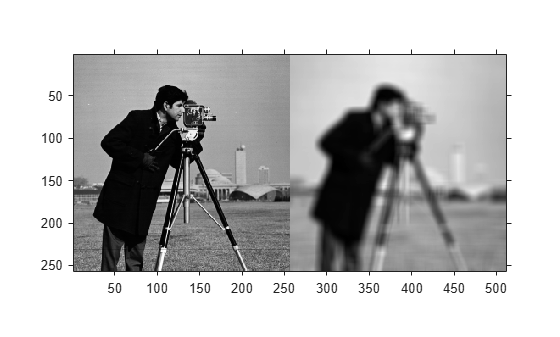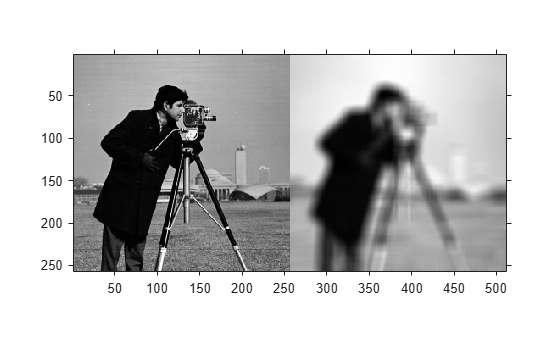# imboxfilt

2-D box filtering of images

## Syntax

``B = imboxfilt(A)``
``B = imboxfilt(A,filterSize)``
``B = imboxfilt(___,Name,Value)``

## Description

example

````B = imboxfilt(A)` filters image `A` with a 2-D, 3-by-3 box filter. A box filter is also called a mean filter.```

example

````B = imboxfilt(A,filterSize)` filters image `A` with a 2-D box filter with size specified by `filterSize`.```

example

````B = imboxfilt(___,Name,Value)` uses name-value pair arguments to control aspects of the filtering.```

## Examples

collapse all

`A = imread('cameraman.tif');`

Perform the mean filtering using an 11-by-11 filter.

`localMean = imboxfilt(A,11);`

Display the original image and the filtered image, side-by-side.

`imshowpair(A,localMean,'montage')``A = imread('cameraman.tif');`

Change the data type of the image to `double` to avoid integer overflow.

`A = double(A);`

Filter image, calculating local area sums, using a 15-by-15 box filter. To calculate local area sums, rather than the mean, set the `NormalizationFactor` parameter to 1.

`localSums = imboxfilt(A, 15, 'NormalizationFactor',1);`

Display the original image and the filtered image, side-by-side.

`imshowpair(A,localSums,'montage')`## Input Arguments

collapse all

Image to be filtered, specified as a numeric array of any dimension. If the input image has more than two dimensions (`ndims(I)>2`), such as for an RGB image, then `imboxfilt` performs box filtering of all 2-D planes along the higher dimensions.

If `A` contains `Infs` or `NaNs`, then the behavior of `imboxfilt` is undefined. This can happen when integral image based filtering is used. To restrict the propagation of `Infs` and `NaNs` in the output, consider using `imfilter` instead.

Data Types: `single` | `double` | `int8` | `int16` | `int32` | `uint8` | `uint16` | `uint32`

Size of box filter, specified as a positive odd integer or 2-element vector of positive, odd integers. If `filterSize` is scalar, then the box filter is square.

Data Types: `single` | `double` | `int8` | `int16` | `int32` | `int64` | `uint8` | `uint16` | `uint32` | `uint64`

### Name-Value Arguments

Specify optional pairs of arguments as `Name1=Value1,...,NameN=ValueN`, where `Name` is the argument name and `Value` is the corresponding value. Name-value arguments must appear after other arguments, but the order of the pairs does not matter.

Before R2021a, use commas to separate each name and value, and enclose `Name` in quotes.

Example: `B = imboxfilt(A,5,'Padding','circular');`

Padding pattern, specified as one of the following values or a numeric scalar. If you specify a scalar value, input image pixels outside the bounds of the image are implicitly assumed to have the scalar value.

ValueDescription
`'circular'`Input image values outside the bounds of the image are computed by implicitly assuming the input image is periodic.
`'replicate'`Input image values outside the bounds of the image are assumed equal to the nearest image border value.
`'symmetric'`Input image values outside the bounds of the image are computed by mirror-reflecting the array across the array border.

Data Types: `single` | `double` | `int8` | `int16` | `int32` | `int64` | `uint8` | `uint16` | `uint32` | `uint64` | `char` | `string`

Normalization factor applied to box filter, specified as a numeric scalar.

The default `'NormalizationFactor'` has the effect of a mean filter — the pixels in the output image are the local means of the image over the neighborhood determined by `filterSize`. To get local area sums, set `'NormalizationFactor'` to `1`. To avoid overflow in such circumstances, consider using double precision images by converting the input image to class `double`.

Data Types: `single` | `double` | `int8` | `int16` | `int32` | `int64` | `uint8` | `uint16` | `uint32` | `uint64`

## Output Arguments

collapse all

Filtered image, returned as a numeric array of the same size as the input image `A`.

## Algorithms

`imboxfilt` performs filtering using either convolution-based filtering or integral image filtering, using an internal heuristic to determine which filtering approach to use.

## Version History

Introduced in R2015b